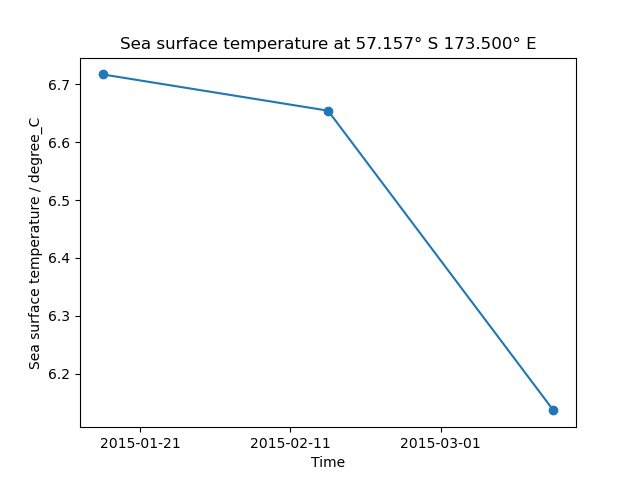# Load a Time Series of Data From the NEMO Model#

This example demonstrates how to load multiple files containing data output by the NEMO model and combine them into a time series in a single cube. The different time dimensions in these files can prevent Iris from concatenating them without the intervention shown here.```import matplotlib.pyplot as plt

import iris
import iris.plot as iplt
import iris.quickplot as qplt
from iris.util import equalise_attributes, promote_aux_coord_to_dim_coord

def main():
# Load the three files of sample NEMO data.
fname = iris.sample_data_path("NEMO/nemo_1m_*.nc")

# Some attributes are unique to each file and must be removed to allow
# concatenation.
equalise_attributes(cubes)

# The cubes still cannot be concatenated because their dimension coordinate
# is "time_counter", which has the same value for each cube.  concatenate
# needs distinct values in order to create a new DimCoord for the output
# cube.  Here, each cube has a "time" auxiliary coordinate, and these do
# have distinct values, so we can promote them to allow concatenation.
for cube in cubes:
promote_aux_coord_to_dim_coord(cube, "time")

# The cubes can now be concatenated into a single time series.
cube = cubes.concatenate_cube()

# Generate a time series plot of a single point
plt.figure()
y_point_index = 100
x_point_index = 100
qplt.plot(cube[:, y_point_index, x_point_index], "o-")

# Include the point's position in the plot's title
lat_point = cube.coord("latitude").points[y_point_index, x_point_index]
lat_string = "{:.3f}\u00B0 {}".format(
abs(lat_point), "N" if lat_point > 0.0 else "S"
)
lon_point = cube.coord("longitude").points[y_point_index, x_point_index]
lon_string = "{:.3f}\u00B0 {}".format(
abs(lon_point), "E" if lon_point > 0.0 else "W"
)
plt.title(
"{} at {} {}".format(
cube.long_name.capitalize(), lat_string, lon_string
)
)

iplt.show()

if __name__ == "__main__":
main()
```

Total running time of the script: ( 0 minutes 0.308 seconds)

Gallery generated by Sphinx-Gallery